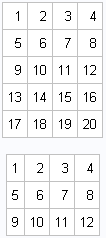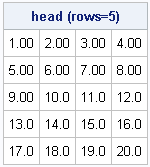Last month I blogged about defining SAS/IML functions that have default parameter values. This language feature, which was introduced in SAS/IML 12.1, enables you to skip arguments when you call a user-defined function.

The same technique enables you to define optional parameters. Inside the function, you can determine whether the optional parameter was specified by using the ISSKIPPED function.

### Print the first few rows of a matrix

For example, suppose you want to implement a module that prints the first few rows of a matrix. By default, you want to print five rows. However, you also want to support printing a specified number of rows. You can implement a module that does this task by using the new 12.1 syntax for specifying default parameter values. The UNIX operating system has a command that called head that prints the first few rows of a file, so I'll use the same name for this module. A first attempt might be as follows:

```proc iml; start head1(x, n=min(5,nrow(x))); /* 1st attempt: one arg with default value */ print (x[1:n,]); finish;   x = shape(1:36, 9); /* test matrix is 9 x 4 */ run head1(x); /* print first 5 rows (default) */ run head1(x, 3); /* print first 3 rows */```The HEAD1 module works as advertise: it prints five rows by default, but also enables you to specify the number of rows. Notice the way that I specified the default value for the second argument (n). In the START statement, I put an equal sign (=) after the name of the argument and then I specified an expression that determines the default value. For this example, the expression contains a call to the MIN function in order to support matrices that have fewer than five rows. In other words, the default value for n depends on the size of x, the first argument. The value for n is determined as follows:

• If the module is called with two arguments, then n is assigned the value of the second specified argument. The expression that computes the default value is ignored.
• If the module is called with one argument, then n is assigned a default value, which is determined by evaluating the expression min(5, nrow(x)). When x has five or more rows, then n = 5. When x has fewer than five rows, n is assigned to be the number of rows in x.

### Specifying a complicated default value

In this example, the default value of the n argument was moderately complicated, but I could still specify it by using a single expression. If the default value requires executing several statements, then you can't specify the value on the START statement. Instead, use an equal sign to specify that the argument is optional, and use the ISSKIPPED function to check whether the argument was specified or whether it should receive a default value, as follows:

```start head2(x, n=); /* 2nd attempt: one optional arg */ if isskipped(n) then do; /* use as many statements as necessary to assign a default value */ n = min(5,nrow(x)); end; print (x[1:n,]); finish;```

### Optional arguments without default values

Suppose that you want to modify the HEAD1 module so that it supports printing a matrix by using a specified SAS format. By default, you want to print the first few matrix rows by using the default SAS/IML format. However, you want to optionally specify a format.

In this scenario, there is no appropriate default value for the format. To specify that an argument is optional (but does not receive a default value), place an equal sign (=) after the argument in the START statement. You can use the ISSKIPPED function to detect whether the optional argument was specified, as follows:

```/* n has a default value; format is optional */ start head(x, n=min(5,nrow(x)), format=); labl = "head (rows=" + strip(char(n)) + ")"; /* construct label */ if isskipped(format) then print (x[1:n,])[label=labl]; /* print with default format */ else do; y = putn(x[1:n,], format); /* apply specified format */ print y[label=labl]; /* print formatted matrix */ end; finish;   run head(x) format="4.2"; /* specify a format */```Inside the HEAD module, the ISSKIPPED function determines at run time whether the third argument was specified. If not, then the PRINT statement prints the first few rows of the matrix. (The default SAS/IML format is used.) If the third argument is specified, then the PUTN statement applies the specified format to the first few rows and prints the result.

Optional arguments can be a powerful tool. Default arguments make it easier to call a function because the user needs to specify only a small number of the possible options to the function. As this example shows, default values can depend on run time values of other arguments. Default arguments are one of my favorite features in SAS/IML 12.1. I hope that you find them as useful as I do!

Share